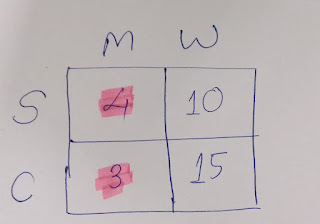# Data Science Simplified

Learning the Machine Learning, in a Human-friendly Way

### The Chi-Square Test Explained with Examples: A Beginner's Guide

Imagine, in a large gathering, people were given an option to buy any one of the two products for free. You want to test if is there any relation between gender and buying patterns.

When variables are independent

You take a random sample of 40 persons: in which there were 10 men and 10 women. You asked them what did they buy? A pen or a pencil?

The cross-tabulated data is shown in the following table:

This type of cross-tabulation is called the contingency table. As you can see, both men and women equally preferred pen and pencil. There were no differences in buying patterns across gender. The chi-squared test statistic in such cases will be not significant.

When variables are not independent

Imagine, instead of pen and pencil, they were given the option to buy either a soft drink or chocolate. You randomly surveyed 52 persons, of which 28 were men and 24 were women. The results are summarized below:

The chi-squared test statistic, in this case, is significant (11.1429,  p-value 0.000844). As you can see, men preferred soft drinks while women liked to buy chocolates. In the 2x2 table, if the diagonal values are higher compared to off-diagonal values, then usually the variables are not independent.
For example, in our example, diagonal values (20 & 18) were higher compared to off-diagonal values of 8 and 6. Hence, the chi-squared test statistic was significant.

Calculation of chi-squared statistic
χ2 = ∑ (observed value - expected value)⋀2/expected value
Expected value = row total* column total /grand total
For the above example, let us calculate the row, column and grand total.
The expected value is row total (26) multiplied by column total (28) divided by grand total (52).
Then let us calculate (observed value - expected value)⋀2/expected value for each cell.
Once we calculate these, let us sum these values to get the chi-squared statistic. The degrees of freedom is (row-1)(column-1) = (2-1)(2-1) =1.
The chi-squared test is used to test independence between variables (which we studied now) and to test the goodness of fit.

• Used as chi-square goodness-of-fit test and the chi-square test for independence
• Easy to compute
• No assumptions about the distribution
• Can be used for nominal scale data (e.g. gender in our example)
Limitations
• Sample size requirements:
For simplicity, it is followed that, if up to 20% (≤ 20%) of expected cell counts are less than 5, then chi-square test can be used. Otherwise, we use Fisher’s exact test (source).

In the following example, you can see that 50% of the expected cell counts are less than 5. Hence, chi-square test is not suitable.50% of expected cell counts are less than 5 - chi-square test not suitable

In summary, the chi-square test is a powerful tool used in social science research, quality control and manufacturing, mainly to test the association between two categorical variables.